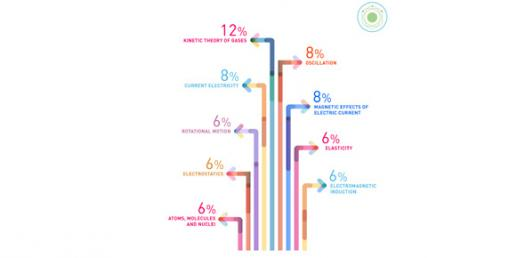# Physics Chapter 22

58 Questions | Total Attempts: 168SettingsPhysics Chapter 22

Related Topics
• 1.
Which force binds atoms together to form molecules?
• A.

Gravitational

• B.

Nuclear

• C.

Electrical

• D.

Centripetal

• E.

None of these

• 2.
The fundamental force underlying all chemical reactions is
• A.

Gravitational

• B.

Nuclear

• C.

Centripetal

• D.

Electrical

• E.

None of these

• 3.
In an electrically neutral atom the number of protons in the nucleus is equal to the number of
• A.

Electrons that surround the nucleus

• B.

Neutrons in the nucleus

• C.

Both of these

• D.

Neither of these

• 4.
A positive ion has more
• A.

Electrons than neutrons

• B.

Electrons than protons

• C.

Protons than electrons

• D.

Protons than neutrons

• E.

Neutrons than protons

• 5.
Strip electrons from an atom and the atom become a
• A.

Positive ion

• B.

Negative ion

• C.

Different element

• 6.
To say that electric charge is quantized is to say that the charge on an object
• A.

May occur in an infinite variety of quantities

• B.

Is a whole number multiple of the charge of one electron

• C.

Will interact with neighboring electric charges

• D.

Can be neither created nor destroyed

• 7.
To say that electric charge is conserved is to say that electric charge
• A.

May occur in an infinite variety of quantities

• B.

Is a whole number multiple of the charge of one electron

• C.

Will interact with neighboring electric charges

• D.

Can be neither created nor destroyed

• 8.
The unit of electric charge, the coulomb, is the charge on
• A.

One electron

• B.

A specific large number of electrons

• 9.
A main difference between gravitational and electric forces is that electrical forces
• A.

Attract

• B.

Repel or attract

• C.

Obey the inverse-square law

• D.

Act over shorter distances

• E.

Are weaker

• 10.
The electrical force between charges is strongest when the charges are
• A.

Close together

• B.

Far apart

• C.

The electric force is constant everywhere

• 11.
The electrical force between charges depends on the
• A.

Magnitude of electric charges

• B.

Separation distance between electric charges

• C.

Both of these

• D.

None of these

• 12.
A transistor is an example of
• A.

A resistor

• B.

A superconductor

• C.

A semiconductor

• D.

A dry cell

• E.

Electrostatic shielding

• 13.
Superconductors are noted for their
• A.

High electric resistance

• B.

Low electric resistance

• C.

Absence of electric resistance

• 14.
Rub electrons from your hair with a comb and the comb becomes
• A.

Negatively charged

• B.

Positively charge

• 15.
An electron and a proton
• A.

Attract each other

• B.

Repel each other

• 16.
Two protons attract each other gravitationally and repel each other electrically. By far the greater is
• A.

The gravitational attraction

• B.

The electrical repulsion

• C.

Neither- they are the same

• 17.
• A.

Attract lightning and guide it to the ground

• B.

Discharge the structure to which it is attached.

• C.

Cancel the electric field within the structure to which it is attached

• D.

Induce within the structure to which it is attached a charge opposite to that of charged clouds overhead

• 18.
To say that an object is electrically polarized is to say
• A.

It is electrically charged

• B.

Its charges have been rearranged

• C.

Its internal electric field is zero

• D.

It is only partially conducting

• E.

It is to some degree magnetic

• 19.
A balloon will stick to a wooden wall if the balloon is charged
• A.

Negatively

• B.

Positively

• C.

Either way

• D.

Neither way

• 20.
When a car is struck by lightning, the resulting electric field inside the car is
• A.

Normally huge, but for brief time

• B.

Normally huge for a time longer than the lightning stroke itself

• C.

Small enough to be safe for an occupant inside

• D.

Zero

• 21.
Electric potential, measured in volts, is the ratio of electric energy to amount of electric
• A.

Current

• B.

Resistance

• C.

Charge

• D.

Voltage

• E.

None of these

• 22.
The electric field inside the dome of a Van de Graaff generator
• A.

Depends on the amount of charge on it

• B.

Depends on its size

• C.

Both of these

• D.

Neither of these

• 23.
An uncharged pitch ball is suspended by a nylon fiber. When a negatively charged rubber rod is brought nearby, without touching it, the pitch ball
• A.

Becomes charged by induction

• B.

Becomes polarized

• C.

Is repelled by the rod

• D.

All of these

• E.

None of these

• 24.
The net charge on a charged capacitor
• A.

Depends on the area of the capacitor plates

• B.

Depends on the distance between the capacitor plates

• C.

Depends on the medium between the capacitor plates

• D.

All of these

• E.

None of these- the net charge is zero

• 25.
Charges on the plates of a charged capacitor reside on the surfaces
• A.

Between the capacitor plates

• B.

Exterior to the capacitor